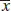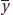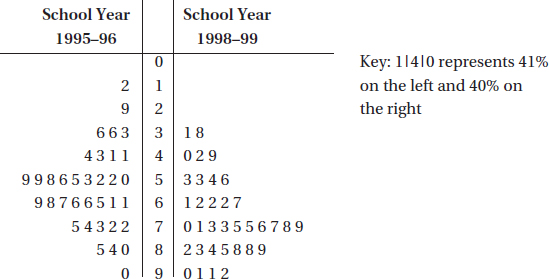# AP Statistics Multiple-Choice Practice Test 36

### Test Information10 questions23 minutes

1. It is estimated that 30% of all cars parked in a metered lot outside City Hall receive tickets for meter violations. In a random sample of 5 cars parked in this lot, what is the probability that at least one receives a parking ticket?

2. Data are collected on income levels x versus number of bank accounts y. Summary calculations give= 32,000; sx = 11,500;= 1.7; sy = 0.4, and r = 0.42. What is the slope of the least squares regression line of number of bank accounts on income level?

3. Given that the sample has a standard deviation of zero, which of the following is a true statement?

4. In one study half of a class were instructed to watch exactly 1 hour of television per day, the other half were told to watch 5 hours per day, and then their class grades were compared. In a second study students in a class responded to a questionnaire asking about their television usage and their class grades.

5. All of the following statements are true for all discrete random variables except for which one?

6. In leaving for school on an overcast April morning you make a judgment on the null hypothesis: The weather will remain dry. What would the results be of Type I and Type II errors?

7. The mean thrust of a certain model jet engine is 9500 pounds. Concerned that a production process change might have lowered the thrust, an inspector tests a sample of units, calculating a mean of 9350 pounds with a z-score of -2.46 and a P-value of 0.0069. Which of the following is the most reasonable conclusion?

8. In a group of 10 third graders, the mean height is 50 inches with a median of 47 inches, while in a group of 12 fourth graders, the mean height is 54 inches with a median of 49 inches. What is the median height of the combined group?

9. A reading specialist in a large public school system believes that the more time students spend reading, the better they will do in school. She plans a middle school experiment in which an SRS of 30 eighth graders will be assigned four extra hours of reading per week, an SRS of 30 seventh graders will be assigned two extra hours of reading per week, and an SRS of 30 sixth graders with no extra assigned reading will be a control group. After one school year, the mean GPAs from each group will be compared. Is this a good experimental design?

10. A study at 35 large city high schools gives the following back-to-back stemplot of the percentages of students who say they have tried alcohol.Which of the following does not follow from the above data?